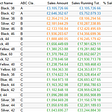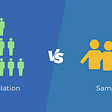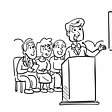# 10 Tips to Improve Your Regression Modeling

## From the book "Regression and Other Stories"

Regression modeling is a powerful tool that can be applied to many events in real life. It's heavily used in many companies on all sorts of business issues.

Regression can be used to explain a certain event, e.g., why sales dropped last month; make predictions, e.g., what sales will be in the next five months; and to make decisions, e.g., should we implement this or that marketing strategy.

The fundamental idea of regression is to understand and estimate the relationship between independent variables (promotions, holidays, advertisements, etc.) and dependent variables (monthly sales).

Doing regression analysis can help us determine what variables impact the monthly sales, answering questions like "Which factors are do we keep and throw away," "how do these factors interact with each other?" etc.

## Regression in the real world

Many statistics textbook you read on regression focuses on math and provide simple examples that aren't realistic. Real-world statistics, however, is complex.

I've been looking for a book that can provide me with insights into how regression is used to do estimations and predictions in the real world.

The search ended when I stumbled upon the book Regression and Other Stories by Professor Andrew Gelman, Jennifer Hill, Aki Vehtari.

Andrew Gelman also writes on the popular blog "Statistical Modeling, Causal Inference, and Social Science," which talks about everything from Causal Inference and Bayesian statistics to Political science and Sports.

The book is awesome as it focuses on practical issues such as missing data and provides a wide range of techniques to solve them.

## Ten tips to improve regression modeling

The appendix section provides ten quick tips to improve your regression modeling, which I found very insightful.

So, in this article, I will be sharing those tips and include some of my takeaways.

Be sure to check out the book's website to further improve your regression game!

Subscribe to our AI NewsletterDeep Grit.
Want to read it first? Check out our latest issue!

# 0. Assumptions of regression analysis

Before we get into the tips, let's make sure we understand the assumptions of the regression model.

In decreasing order of importance :

1. Validity — The data you are analyzing should map to the research question you are trying to answer; the model should include all relevant predictors and should generalize to cases to which it will be applied
2. Representativeness — The goal of the model is to make inferences about a larger population, so it's essential that the sample is representative of the population
3. Additivity and linearity — The most important mathematical assumption of a linear regression model is that "its deterministic component is a linear function of the separate predictors." → `y=B0 + B1x1 + B2x2 + ...`
4. Independence of errors — Simple linear regression assumes errors from the prediction line are independent (violated in time series, spacial, and multilevel settings)
5. Equal variance of errors — Unequal error variance (a fan pattern in the residual plot) causes issues for probabilistic prediction, but in most cases is a minor issue.
6. Normality of Errors — Distribution of error terms is relevant when predicting individual data points but barely important for estimating the regression line.

# 1. Think about variation and replication

We are taught in statistics class about the importance of the variation in the error term, also known as residual variance.

However, variance is also important for the model in general.

Variation is central to regression modeling, and not just in the error term

It's beneficial to fit the model to various datasets that the model has not seen before, as this produces variance in the relationship between the variables.

This is more useful than only having the standard error from one study, as it gives a sense of variation across different problems.

Another important aspect is replication, which the book notes as an ideal replication, "performing all the steps of a study from scratch, not just increasing sample size and collecting more data within an existing setting."

Doing replication from scratch allows you to capture variation based on different aspects of data collection and measurement, breaking away from the bubble of results seen in a single study.

# 2. Forget about statistical significance

p-values are painted to be the golden rule of determining whether a test has significant results or not, but `p ≤ 0.05` doesn't tell the full story.

— Regression and Other Stories

The book states three key reasons to forget about statistical significance:

## 1. If one discretizes results based on significance tests, you are throwing away information.

Why? — "Measures of significance such as p-values are noisy, and it is misleading to treat an experiment as a success or failure based on a significance test."

## 2. There are no true zeroes (for real-world problems)

Why? — "No true populations are identical, and anything that plausibly could have an effect will not have an effect that is exactly zero."

## 3. Comparisons and effects vary by context

Why? — Having a confidence interval that excludes zero doesn't tell us something useful about future differences.

# 3. Graph the relevant and not the irrelevant

Graphing is one of the most crucial steps in any analysis.

The goal of any graph is communication to self or others

The first step is to always display raw data (EDA), where the goal is to see things you did not expect or even know to look for.

But this begs the question: What is relevant to visualize and not?

## 1. Graph the fitted model

A table of regression coefficients does not tell the same story as the visualizations and graphs.

You can graph the fitted models a couple of ways:

• overlaying data plots to understand model fit
• graph sets of estimated parameters
• plot predicted datasets and compare them visually to actual data

Find examples of these in the book in C10 and C11.

## 2. Graph the data

Real-world data is high in dimension and complex.

It's crucial to make many different graphs of the data and look at the model from different angles.

Instead of single images, use a series of graphs and tell a story through the visualizations.

What is irrelevant?

Many statistics textbooks and classes focus strongly on plots for raw data and regression diagnostics (QQ plot, residual variance)

Although they are relevant when evaluating the use of the model for predicting individual data points, they're not useful for satisfying the assumptions of representativeness, validity, additivity, linearity, etc., in regression.

Rule: Be prepared to explain any graph you show

# 4. Interpret regression coefficients as comparisons

This tip changed how I view regression coefficients.

Take this linear regression equation as an example

`salary = -20 + 0.6 * height + 10.6 * male + error`
where salary is per \$1k and height is in inches
— from page 84 of Regression and Other Stories

It might seem natural to report that the estimated effect of height is 0.6 or 600\$ in this case.

However, it's not appropriate to see them as "effects", as that would suggest that if we increase someone's height by one inch, their earnings will increase by en estimated amount of \$600.

What our model is really telling us is that taller people in our sample have higher earnings on average.

The correct interpretation is — "under the fitted model, the average difference in earnings, comparing two people of the same sex but one inch different in height is \$600."

From the data alone, a regression only tells us about comparisons between individuals, not about changes within individuals

Benefits of thinking of regression as comparisons:

## 1) interpretation as a comparison is always available

Comparisons can be said as the description of the model and do not require any causal assumptions.

## 2) Complicated regressions = built from simpler models

We can consider more complicated regressions as built up from simpler models, starting with simple comparisons and adding adjustments.

## 3) Comparisons also works in the special case of causal inference

The comparative interpretation also works in the special case of causal inference, where we can consider comparisons between the same individual receiving two different levels of a treatment.

# 5. Understand statistical methods using fake-data simulation

Fake-data simulation — Simulate fake data given an assumed generative model to evaluate the properties of statistical methods and procedures being used
Generative models — A model that includes the distribution of the data itself, and tells you how likely a given example is.

Why use fake-data simulation? 4 reasons.

## 1) It helps to increase our understanding of the application

The decisions made in constructing a simulated world based on the model can clarify the following:

• How large a treatment effect could we realistically expect to see
• how large are the interactions we want to consider
• what might be the correlation between pre-test and post-test, and so forth.

## 2) It's a general way to study the properties of statistical methods under repeated sampling.

Put the simulation and inference into a loop, and you can see how close the model's estimates and predictions are to the assumed true values.

Here it can make sense to simulate from a process that includes features not included in the model you will use to fit the data — but, again, this can be a good thing in that it forces you to consider assumptions that might be violated.

## 3) It's a way to debug your code.

With large samples or small data variance, your fitted model should be able to recover the true parameters.

If it can't, you may have a coding problem or a conceptual problem, where your model is not doing what you think it is doing.

It can help in such settings to plot the simulated data overlaid with the assumed and fitted models.

## 4) It's necessary for collecting new data

You need fake-data simulation if you want to design a new study and collect new data with some reasonable expectation of what you might find.

# 6. Fit many models

It's generally good to start with a simple model to understand how well the data fits with the models.

You can also start with a complex model, drop things out and slowly move to a simpler model.

Realistically, you don’t know what model you want, so it’s good to fit models quickly.

Another great tip is to keep track of the models you have fit. This helps you understand your data and protect yourself from biases that can arise when you have many ways of analyzing data.

Thus, make it a habit to record all the procedures you've done and report the results from all relevant models.

# 7. Set up a computational workflow

Faster and more reliable computation = better statistical workflow

if you can fit models faster, you can fit more models and better understand both data and model.

There are two approaches:

## 1. Data subsetting

A trick to speed up computations is to break a large dataset into subsets and analyze each separately.

Advantage — Faster computation while allowing you to explore the data by trying out more models. Separate analysis that is well executed can also reveal variation across subsets.

Disadvantage — (1) Inconvenient to partition data, perform analysis, and summarize the results. (2) Separate analysis may not be as accurate as putting all the data together in a single analysis

Solution: Use multilevel modeling to subset without losing inferential efficiency.

## 2. Fake-data and predictive simulation

Fake data and predictive simulation effectively diagnose problems in the code or the model fit.

Predictive simulation — Using Bayesian generalized linear models to obtain simulations to make probabilistic predictions

## How?

(1) Use fake-data simulation to check the correctness of the code
(2) Use predictive simulation to compare the data to the fitted model's prediction.

# 8. Use transformations

Two transformations that are commonly used are:

## 1. Log-transformation

You always hear about log transforming to help deal with skewness.

However, it's more important for satisfying the assumptions of validity, additivity, and linearity.

For example, if additivity is violated, i.e., instead of `y = B0 + B1x1` you have, a log transformation would give you `log y = log a + log b + log c`

It's also common for linearity to be violated, i.e., nonlinear functions in medical fields where a health measure declines with higher ages, they can be solved by getting the reciprocal of the predictor `1/x` or `log(x)` or with nonlinear functions like splines or Gaussian process

## 2. Standardization

Standardizing is useful to keep all the data within a certain scale or range so that statistics can be directly interpreted and scaled.

The above transformations were univariate. In addition to that, you can create interactions and engineer new features by combining inputs.

# 9. Do causal inference in a targeted way, not as a byproduct of a large regression

This tip warns against assuming that a comparison or regression coefficient can be interpreted causally.

The book states that if you are interested in causal inference, consider your treatment variable carefully and use the tools in the book (Chapters 18–21) to address the challenges of balance and overlap when comparing treated and control units to estimate a causal effect and its variation across the population.

It continues to explain that even if you are using a natural experiment or identification strategy, it is important to compare treatment and control groups and adjust for pre-treatment differences.

When considering several causal questions, it can be tempting to set up a single large regression to answer them all at once; however, this is not appropriate in observational settings (including experiments in which certain conditions of interest are observational).

# 10. Learn methods through live examples

This is my favorite tip, and what I think is the best tip, as it leaves the reader with an action plan.

If you want to learn and apply complicated statistics methods, apply them to problems you care about.

First, gather data on the examples using the right data-collection procedures.

This requires being aware of the population of interest.

Before you dive into the analysis, determine the larger goals of your data collection and analysis, be specific about what you want to achieve and whether you can achieve it with the data you have.

Then, develop statistical understanding of the data through simulation and visualizations.

That's all for this article, be sure to check out the book for code examples and explanations that go in-depth into each of these tips 👇

Also, feel free to leave your thoughts on these tips in the comments!

Be sure to follow the bitgrit Data Science Publication to keep updated!

Want to discuss the latest developments in Data Science and AI with other data scientists? Join our discord server!

--

--

--

## More from bitgrit Data Science Publication

We’re democratizing AI with our online competition platform — bitgrit.net. On our publication, we publish only high-quality data science-related topics. Become a writer by emailing us at: info@bitgrit.net

## Applying VACUUM to Data## What promotion to send to your customer? Hear what data has to say.## ABC Classification Analysis## What does Everyone Buy on Amazon?## INFORMATION## Using Proof of Stake (PoS) Consensus Algorithm on the ERC20 Platform for a Native School Coin —…## Diving into Gremlin queries in Azure Cosmos DB## Photo Essay: MLK Day Seattle Rally and March## Benedict Neo

+1.5M views | Connect 👉 https://linkedin.com/in/benedictneo/

## Machine learning to predict our wins on Brawlhalla — Part Two: Predictive Analysis (with…## Difference between Population Data and Sample Data.## No Data Scientist is the same!## Project Management in Data Science using SEMMA